# Learning Objectives

• Explain the dependencies between hardware and software
• Describe the form and the function of computer programming languages
• Create, modify, and explain computer programs following the input/process/output pattern.
• Form valid Python identifiers and expressions.
• Write Python statements to output information to the screen, assign values to variables, and accept information from the keyboard.
• Read and write programs that process numerical data and the Python math module.
• Read and write programs that process textual data using built-in functions and methods.

# Computer Hardware Architecture

Before we start learning a programming language to give instructions to computers to develop software, we need to learn about how computers are built. If you were to take apart your computer or cell phone and look deep inside, you would find the following parts: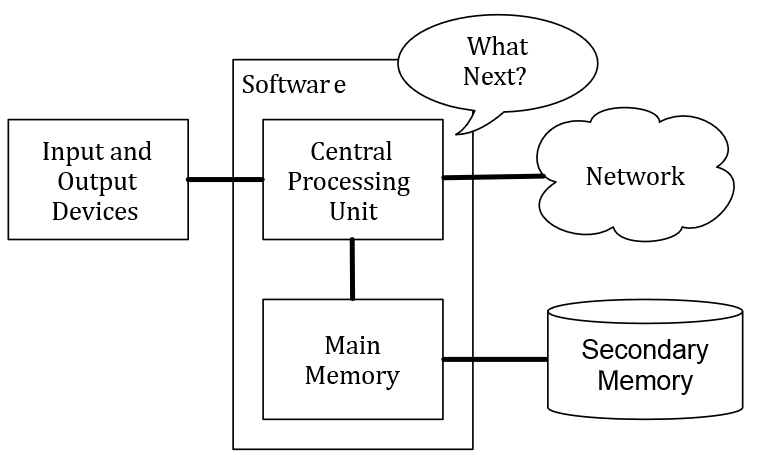Figure 10: Computer Hardware Architecture

The high-level definitions of these parts are as follows:

• The Central Processing Unit (or CPU) is the part of the computer that is built to be obsessed with “what is next?” If your computer is rated at 3.0 Gigahertz, it means that the CPU will ask “What next?” three billion times per second.
• The Main Memory is used to store information that the CPU needs in a hurry. The main memory is nearly as fast as the CPU. But the information stored in the main memory vanishes when the computer is turned off.
• The Secondary Memory is also used to store information, but it is much slower than the main memory. The advantage of the secondary memory is that it can store information even when there is no power to the computer. Examples of secondary memory are disk drives or flash memory (typically found in USB sticks and portable music players).
• The Input and Output Devices are simply our screen, keyboard, mouse, microphone, speaker, touchpad, etc. They are all of the ways we interact with the computer.
• These days, most computers also have a Network Connection to retrieve information over a network. We can think of the network as a very slow place to store and retrieve data that might not always be “up”. So in a sense, the network is a slower and at times unreliable form of Secondary Memory.

While most of the detail of how these components work is best left to computer builders, it helps to have some terminology so we can talk about these different parts as we write our programs.

As a programmer, your job is to use and orchestrate each of these resources to solve the problem that you need to solve and analyze the data you get from the solution. As a programmer you will mostly be “talking” to the CPU and telling it what to do next. Sometimes you will tell the CPU to use the main memory, secondary memory, network, or the input/output devices.

# Digital Computing: It’s All about 0’s and 1’s

It is essential that computer hardware be reliable and error free. If the hardware gives incorrect results, then any program run on that hardware is unreliable. The key to developing reliable systems is to keep the design as simple as possible. In digital computing, all information is represented as a series of digits or electronic symbols which are either “on” or “off” (similar to a light switch). These patterns of electronic symbols are best represented as a sequence of zeroes and ones, digits from the binary (base 2) number system.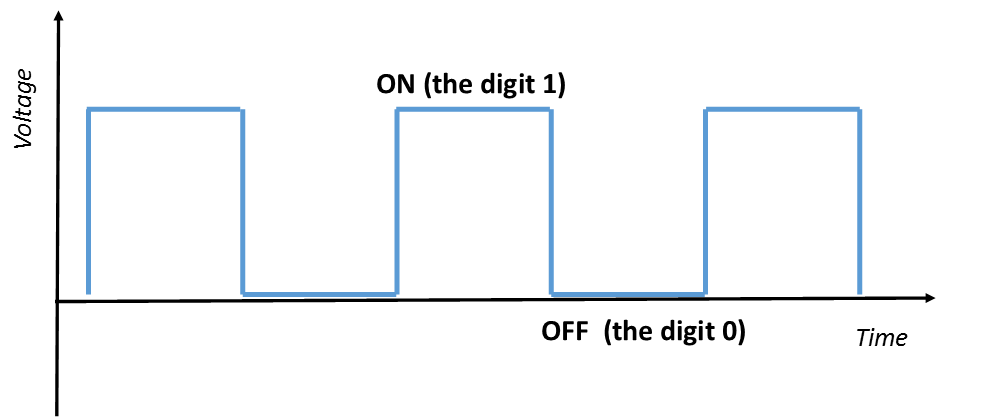Figure 11: Digital Representation

The term bit stands for binary digit. Therefore, every bit has the value 0 or 1. A byte is a group of bits operated on as a single unit in a computer system, usually consisting of eight bits. Although values represented in base 2 are significantly longer than those represented in base 10, binary representation is used in digital computing because of the resulting simplicity of hardware design. For example, the decimal number `485` is represented in binary as `111100101`.

# Operating Systems—Bridging Software and Hardware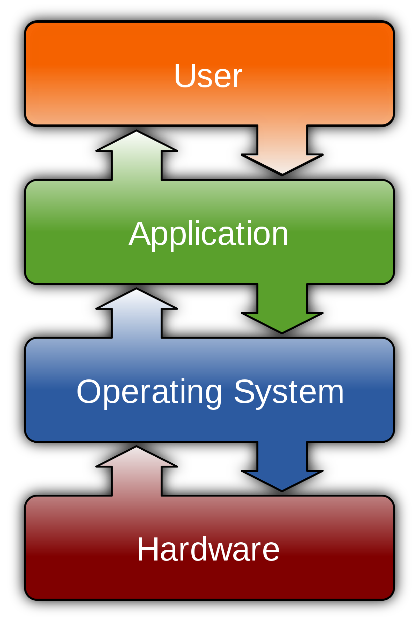An operating system is software that has the job of managing and interacting with the hardware resources of a computer. Because an operating system is intrinsic to the operation a computer, it is referred to as system software.

An operating system acts as the “middle man” between the hardware and executing application programs (see Figure 12). For example, it controls the allocation of memory for the various programs that may be executing on a computer. Operating systems also provide a particular user interface. Thus, it is the operating system installed on a given computer that determines the “look and feel” of the user interface and how the user interacts with the system, and not the particular model computer. 

# Software Development Tools

It can be very cumbersome, error prone, and time consuming to converse with a computer using only zeros and ones. Numerical machine code (computer code using only zeros and ones) does exist but is rarely used by programmers. For that reason most people program using a “higher-level” programming language which use words and symbols that are easier for humans to manage than binary sequences. Tools exist that automatically convert a higher-level description of what is to be done into the required lower-level, machine code. Higher-level programming languages like Python allow programmers to express solutions to programming problems in terms that are much closer to a natural language like English. Some examples of the more popular of the hundreds of higher-level programming languages that have been devised over the past 60 years include FORTRAN, COBOL, Lisp, Haskell, C++, Perl, C , Java, and C#. Most programmers today, especially those concerned with high-level applications, usually do not worry about the details of underlying hardware platform and its machine language.

Sample numerical machine code:
`001010001110100100101010000001111`
`11100110000011101010010101101101`

Fortunately, higher-level programming languages provide a relatively simple structure with very strict rules for forming statements, called the programming language syntax, which can express a solution to any problem that can be solved by a computer.

Consider the following program fragment written in the Python programming language:

`subtotal = 25`
`tax = 3`
`total = subtotal + tax`

While these three lines (three statements) do constitute a proper Python program, they are more likely a small piece of a larger program. The lines of text in this program fragment look similar to expressions in algebra. We see no sequence of binary digits. Three words,` subtotal, tax, and total`, called variables, represent information. In programming, a variable represents a value stored in the computer’s memory. Instead of some cryptic binary instructions meant only for the CPU, we see familiar-looking mathematical operators (= and +). Since this program is expressed in the Python language, not machine language, no computer processor (CPU) can execute the program directly. A program called an interpreter translates the Python code into machine code when a user runs the program. The higher-level language code is called the source code. The corresponding machine language code is called the target code. The interpreter translates the source code into the target machine language.

The beauty of higher-level languages is this: the same Python source code can execute on different target platforms. The target platform must have a Python interpreter available, but multiple Python interpreters are available for all the major computing platforms. The human programmer therefore is free to think about writing the solution to the problem in Python, not in a specific machine language.

Programmers have a variety of tools available to enhance the software development process. Some common tools include:

• Editors. An editor allows the programmer to enter the program source code and save it to files. Most programming editors increase programmer productivity by using colors to highlight language features. The syntax of a language refers to the way pieces of the language are arranged to make well-formed “sentences.” To illustrate, the sentence

The tall boy runs quickly to the door.

uses proper English syntax. By comparison, the sentence

Boy the tall runs door to quickly the.

is not correct syntactically. It uses the same words as the original sentence, but their arrangement does not follow the rules of English.

Similarly, programming languages have strict syntax rules that programmers must follow to create well-formed programs. Only well-formed programs are acceptable for translation into executable machine code. Some syntax-aware editors can use colors or other special annotations to alert programmers of syntax errors during the editing process.

• Compilers. A compiler translates the source code to target code. The target code may be the machine language for a particular platform or embedded device. The target code could be another source language; for example, the earliest C++ compiler translated C++ into C, another higher-level language. The resulting C++ code was then processed by a C++ compiler to produce an executable program. (C++ compilers today translate C++ directly into machine language.) Compilers translate the contents of a source file and produce a file containing all the target code. Popular compiled languages include C, C++ , Java, C#.
• Interpreters. An interpreter is like a compiler, in that it translates higher-level source code into target code (usually machine language). It works differently, however. While a compiler produces an executable program that may run many times with no additional translation needed, an interpreter translates source code statements into machine language each time a user runs the program. A compiled program does not need to be recompiled to run, but an interpreted program must be reinterpreted each time it executes. The interpreter in essence reads the code one line at a time. In general, compiled programs execute more quickly than interpreted programs because the translation activity occurs only once. Interpreted programs, on the other hand, can run as is on any platform with an appropriate interpreter; they do not need to be recompiled to run on a different platform. Python, for example, is used mainly as an interpreted language, but compilers for it are available. Interpreted languages are better suited for dynamic, explorative development which many people feel is ideal for beginning programmers. Popular scripting languages include Python, Ruby, Perl, and, for web browsers, Javascript.

The Python interpreter is written in a high-level language called “C”. You can look at the actual source code for the Python interpreter by going to www.python.org and working your way to their source code. So Python is a program itself and it is compiled into machine code. When you install Python on your computer, you copy a machine-code copy of the translated Python program onto your system. In Windows, the executable machine code for Python itself is likely in a file with a name like:

`C:\Python35\python.exe`

• Debuggers. A debugger allows a programmer to more easily trace a program’s execution in order to locate and correct errors in the program’s implementation. With a debugger, a developer can simultaneously run a program and see which line in the source code is responsible for the program’s current actions. The programmer can watch the values of variables and other program elements to see if their values change as expected. Debuggers are valuable for locating errors (also called bugs) and repairing programs that contain errors. (See the Debugging Section in this Unit for more information about programming errors.)
 COMPILER INTERPRETER Compiler takes an entire program as input. It works on the complete program at once. Interpreter takes a single statement at a time as input. It works line by line. It generates Intermediate Object code (machine code). It doesn’t generate Intermediate code (machine code). It executes Conditional control statements faster than Interpreter. It takes large amount of time to analyze the source code but the overall execution time is comparatively faster. It executes Conditional control statements much slower than Compiler. In general, the overall execution time is slower. More memory required (since Object Code is generated). Memory requirement is less, hence more memory efficient. It doesn’t generate intermediate Object Code. Compiled program doesn’t need to be compiled every time. Every time higher level program is converted into lower level program. Errors are displayed after entire program is checked. Hence debugging is comparatively hard. Errors are displayed for every instruction interpreted (if any). Continues translating the program until the first error is met, in which case it stops. Hence debugging is easier. Programming languages that use compilers are COBOL, C, C++ . Programming languages that use interpreter are Visual Basic Script, Ruby, Perl.

Table 1: Compiler Vs Interpreter

Many developers use integrated development environments (IDEs). An IDE includes editors, debuggers, and other programming aids in one comprehensive program. Python IDEs include Wingware, PyCharm,, and IDLE.

Despite the wide variety of tools (and tool vendors’ claims), the programming process for all but trivial programs is not automatic. Good tools are valuable and certainly increase the productivity of developers, but they cannot write software. There are no substitutes for sound logical thinking, creativity, common sense, and, of course, programming experience.

# Learning Programming with Python

Guido van Rossum created the Python programming language in the late 1980s. He named the language after the BBC show “Monty Python’s Flying Circus”. In contrast to other popular languages such as C, C++ , Java, and C#, Python strives to provide a simple but powerful syntax.

Python is used for software development at companies and organizations such as Google, Yahoo, Facebook, CERN, Industrial Light and Magic, and NASA. It is especially relevant in developing information science applications such as ForecastWatch.com which uses Python to help meteorologists, online travel sites, airline reservation systems, university student record systems, air traffic control systems among many others. Experienced programmers can accomplish great things with Python, but Python’s beauty is that it is accessible to beginning programmers and allows them to tackle interesting problems more quickly than many other, more complex languages that have a steeper learning curve.

Python has an extensive Standard Library which is a collection of built-in modules, each providing specific functionality beyond what is included in the “core” part of Python. (For example, the math module provides additional mathematical functions. The random module provides the ability to generate random numbers). Additionally the Standard Library can help you do various things involving regular expressions, documentation generation, databases, web browsers, CGI, FTP, email, XML, HTML, WAV files, cryptography, GUI (graphical user interfaces), among other things.

More information about Python, including links to download the latest version for Microsoft Windows, Mac OS X, and Linux, can be found in Appendix A of this book and also at http://www.python.org .

In late 2008, Python 3.0 was released. Commonly called Python 3, the current version of Python, VERSION 3.0, is incompatible with earlier versions of the language. Many existing books and online resources cover Python 2, but more Python 3 resources now are becoming widely available. The code in this book is based on Python 3.

This book does not attempt to cover all the facets of the Python programming language. The focus here is on introducing programming techniques and developing good habits and skills. To that end, this approach avoids some of the more obscure features of Python and concentrates on the programming basics that easily transfer directly to other programming languages.

The goal is to turn you into a person who is skilled in the art of programming. In the end you will be a programmer – perhaps not a professional programmer, but at least you will have the skills to look at a data/information analysis problem and develop a program to solve the problem.

In a sense, you need two skills to be a programmer:

• First, you need to know the programming language (Python) – you need to know the vocabulary and the grammar (the syntax). You need to be able to spell the words in this new language properly and know how to construct well-formed “sentences” in this new language.
• Second, you need to “tell a story”. In writing a story, you combine words and sentences to convey an idea to the reader. There is a skill and art in constructing the story, and skill in story writing is improved by doing some writing (practice) and getting some feedback. In programming, our program is the “story” and the problem you are trying to solve is the “idea”.

Once you learn one programming language such as Python, you will find it much easier to learn a second programming language such as JavaScript or C++ . Other programming languages have very different vocabulary and grammar (syntax) but the problem-solving skills will be the same across all programming languages.

You will learn the “vocabulary” and “sentences” (the syntax) of Python pretty quickly. It will take longer for you to be able to write a coherent program to solve a new problem. We learn programming much like we learn writing. We start by reading and explaining programs, then we write simple programs, and then we write increasingly complex programs over time. At some point you “get your muse” and see the patterns on your own and can see more naturally how to take a problem and write a program that solves that computational problem. And once you get to that point, programming becomes a very pleasant and creative process.

We start with the vocabulary and structure of Python programs. Be patient as the simple examples remind you of when you started reading for the first time.

# Writing a Python Program

The text that makes up a Python program has a particular structure. The syntax must be correct, or the interpreter will generate error messages and not execute the program. This section introduces Python by providing a simple example program.

A program consists of one or more statements. A statement is an instruction that the interpreter executes.

The following statement invokes the print function to display a message:

`print("This is a simple Python program")`

We can use the statement in a program. Figure 13 (`simple.py`) is an example of a very simple Python program that does something: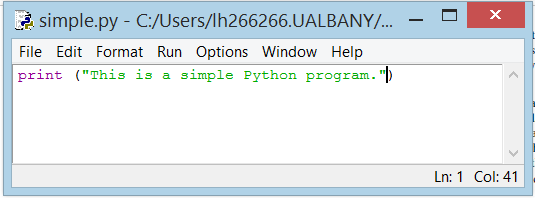Figure 13: Listing of simple.py

IDLE is Python’s Integrated Development and Learning Environment (IDE) and is included as part of the Python Standard Library which is distributed with Python 3 (See Appendix A). IDLE is the standard Python development environment. Its name is an acronym of “Integrated DeveLopment Environment”. It works well on both Unix and Windows platforms.

IDLE has a Python Shell window, which gives you access to the Python interactive mode. It also has a file editor that lets you create and edit existing Python source files. The file editor was used to write the `simple.py` program.

The way you launch IDLE depends on your operating system and how it was installed. Figure 13 shows a screenshot of IDLE running on a Windows 8.1 computer. The IDE consists of a simple menu bar at the top. Other Python IDEs are similar in appearance.

To begin entering our program, we just type in the Python statements. To start a new program, choose the New File item from the File menu. This action produces a new editor pane for a file named `Untitled` as shown in Figure 14 below.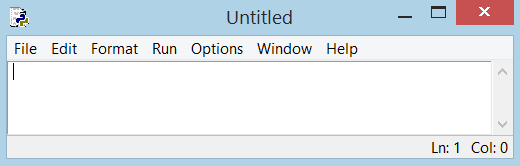Figure 14: New File Editor Window

We now are ready to type in the code that constitutes the program.

`print("This is a simple Python program")`

Next we will save the file. The menu sequence FileSave or File Save As, produces the dialog box shown in Figure 15 that allows us to select a folder and filename for our program. You should be sure all Python programs are saved with a `.py` extension. If “Save as Type: Python files” is available there is no need to add the `.py` extension as it will automatically be saved as a `.py` file (see Figure 15).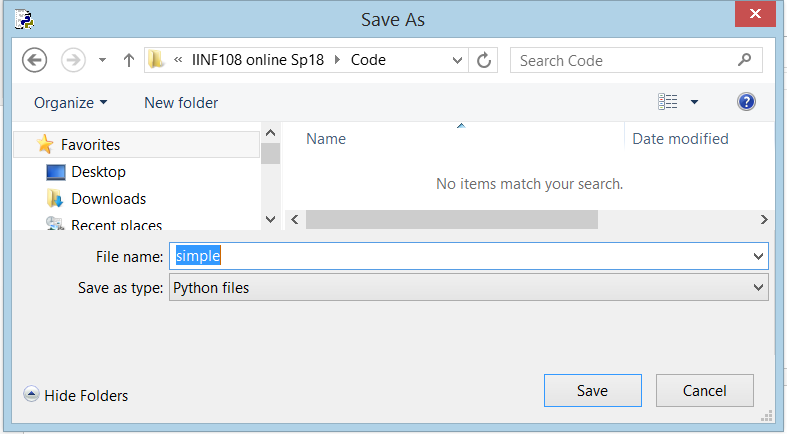Figure 15: Save Python File, Option 1

If you are using a different text editor select “Save as Type: All files” and add the `.py` extension (see Figure 16).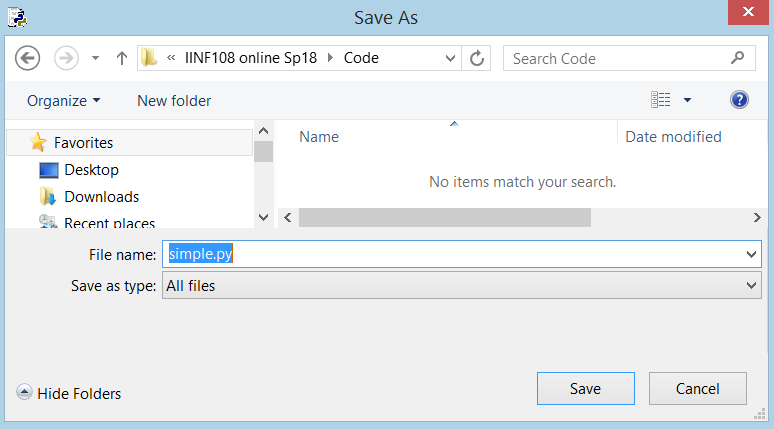Figure 16: Save Python File, Option 2

We can run the program by selecting the menu sequence Run –> Run Module or use the shortcut key F5. A new window labeled Python Shell will display the program’s output. Figure 17 shows the results of running the program.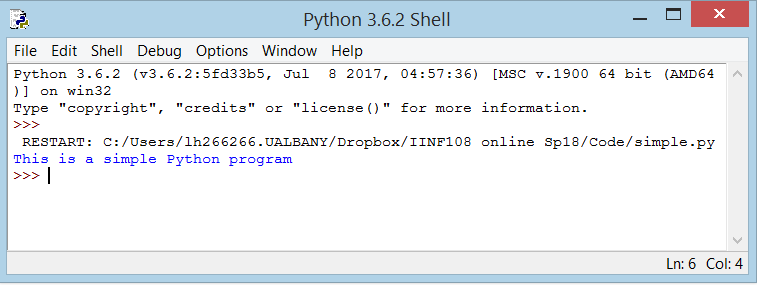Figure 17: Program simple.py Output

This program contains one Python statement. A statement is a command that the interpreter executes. This statement prints the message `This is a simple Python program in the Python Shell window`. A statement is the fundamental unit of execution in a Python program. Statements may be grouped into larger chunks called blocks, and blocks can make up more complex statements (e.g. the selection structure or the iterative structure we saw in the last Unit). The statement `print("This is a simple Python program")` makes use of a built in function named `print`. Python has a variety of different kinds of statements that we can use to build programs, and the sections that follow explore these various kinds of statements.

Note to Reader: In the context of programming, a function is a named sequence of statements that performs a computation. The name of the function here is `print.` The expression in parentheses is called the argument of the function. The result, for this function, is the string of characters in quotes (i.e. the ‘message’) of the argument.It is common to say that a function “takes” an argument and “returns” a result. The result is called the return value.

When you type a statement on the command line in the Shell window and select the Enter key, Python executes it. Statements alone don’t produce any result.

# The Python Interactive Shell

We created the program in Figure 13 (simple.py) and submitted it to the Python interpreter for execution. We can interact with the interpreter directly, typing in Python statements and expressions for immediate execution. As we saw in Figure 17, the IDLE window labeled Python Shell is where the executing program directs its output. We also can type commands into the Python Shell window, and the interpreter will attempt to execute them. Figure 18 shows how the interpreter responds when we enter the program statement directly into the Shell. The interpreter prompts the user for input with three greater-than symbols (>>>). This means the user typed in the text on the line prefixed with >>>. Any lines without the >>> prefix represent the interpreter’s output, or feedback, to the user. We will find Python’s interactive interpreter invaluable for experimenting with various language constructs.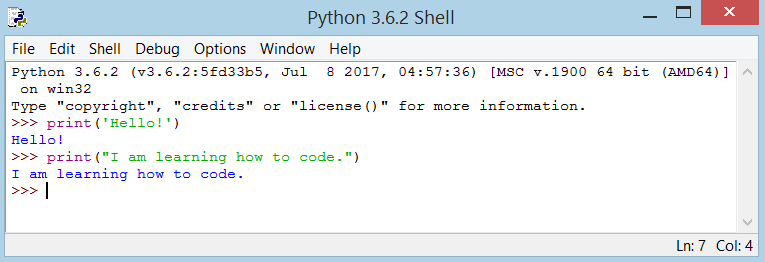Figure 18: Executing Individual Commands in the Python Shell

We can discover many things about Python without ever writing a complete program. We can execute the interactive Python interpreter directly from the command line in the Python Shell. The interpreter prompt (>>>) prefixes all user input in the interactive Shell. Lines that do not begin with the >>> prompt represent the interpreter’s response.If you try to enter each line one at a time into the interactive Shell, the program’s output will be intermingled with the statements you type. In this case the best approach is to type the program into an editor, save the code you type to a file, and then execute the program. Most of the time we use an editor to enter and run our Python programs. The interactive interpreter is most useful for experimenting with small snippets of Python code.

The print() function always ends with an invisible “new line” character ( \n ) so that repeated calls to print will all print on a separate line each. To prevent this newline character from being printed, you can specify that it should end with a blank:
```print('a', end='') print('b', end='')```
Output is:
`ab`
Or you can end with a space:
```print('a', end=' ') print('b', end=' ')print('c')```
Output is:
`a b`
Or you can end with a space:
```print('a', end=' ') print('b', end=' ') print('c')```
Output is:
`a b c`

# Debugging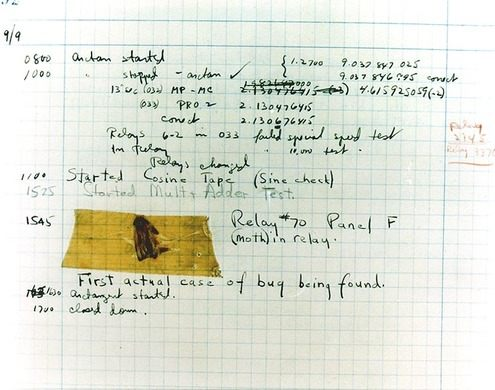Figure 19: First Computer Bug (Image ©Courtesy of the Naval Surface Warfare Center, Dahlgren, VA., 1988. NHHC Collection)

Programming is a complex process, and because it is done by human beings, it often leads to errors. Programming errors are called bugs and the process of tracking them down and correcting them is called debugging.

The story behind this term dates back to September 9, 1947, when Harvard’s Mark II Aiken Relay computer was malfunctioning. After rooting through the massive machine to find the cause of the problem, Admiral Grace Hopper, who worked in the Navy’s engineering program at Harvard, found the bug. It was an actual insect. The incident is recorded in Hopper’s logbook alongside the offending moth, taped to the logbook page: “15:45 Relay #70 Panel F (moth) in relay. First actual case of bug being found.”

Three kinds of errors can occur in a program: syntax errors, runtime errors, and semantic errors. It is useful to distinguish between them in order to track them down more quickly.

### Syntax errors

Python can only execute a program if the program is syntactically correct; otherwise, the process fails and returns an error message. Syntax refers to the structure of a program and the rules about that structure. For example, in English, a sentence must begin with a capital letter and end with a period.

this sentence contains a syntax error.
So does this one

For most readers, a few syntax errors are not a significant problem, which is why we can read the poetry of E. E. Cummings without problems. Python is not so forgiving. If there is a single syntax error anywhere in your program, Python will display an error message and quit, and you will not be able to run your program. Early on, you will probably spend a lot of time tracking down syntax errors. As you gain experience, though, you will make fewer errors and find them faster.

### Runtime errors

The second type of error is a runtime error, so called because the error does not appear until you run the program. These errors are also called exceptions because they usually indicate that something exceptional (and bad) has happened.

Runtime errors are rare in the simple programs you will see in the first Units, so it might be a while before you encounter one.

### Semantic errors

The third type of error is the semantic error. If there is a semantic error in your program, it will run successfully, in the sense that the computer will not generate any error messages and quit, but it will not do the right thing. It will do something else. Specifically, it will do what you told it to do.

The problem is that the program you wrote is not the program you wanted to write. The meaning of the program (its semantics) is wrong. Identifying semantic errors can be tricky because it requires you to work backward by looking at the output of the program and trying to figure out what it is doing. The test cases we generated in UNIT #1 helps programmers fix semantic errors.

### Experimental debugging

One of the most important skills you will acquire is debugging. Although it can be frustrating, debugging is one of the most intellectually rich, challenging, and interesting parts of programming.

In some ways, debugging is like detective work. You are confronted with clues, and you have to infer the processes and events that led to the results you see.

Debugging is also like an experimental science. Once you have an idea what is going wrong, you modify your program and try again. If your hypothesis was correct, then you can predict the result of the modification, and you take a step closer to a working program. If your hypothesis was wrong, you have to come up with a new one.

For some people, programming and debugging are the same thing. That is, programming is the process of gradually debugging a program until it does what you want. The idea is that you should start with a program that does something and make small modifications, debugging them as you go, so that you always have a working program.

# The Basics of Python Programming

Just printing a single sentence is not enough, is it? You want to do more than that – you want to take some input, manipulate it and get something out of it. We can achieve this in Python using constants and variables, and we’ll learn some other concepts as well in this section.

Comments are any text to the right of the # symbol and is mainly useful as notes for the reader of the program.

For example:

`print('hello world') # Note that print is a function`

OR

`# Note that print is a function`
`print('hello world')`

Use as many useful comments as you can in your program to:

• explain assumptions
• explain important decisions
• explain important details
• explain problems you’re trying to solve
• explain problems you’re trying to overcome in your program, etc.

Code tells you how, comments should tell you why.

This is useful for readers of your program so that they can easily understand what the program is doing. Consider the programmer, newly hired, who has been assigned the job of maintaining a 2000 line-of-code program. Without comments the codes can be very difficult to understand let alone maintain.

### Literal Constants

An example of a literal constant is a number like `5` , `1.23` , or a string like `'This is a string'` or `"It's a string!"` (String literals must be in quotes).

It is called a literal because it is literal – you use its value literally. The number 2 always represents itself and nothing else – it is a constant because its value cannot be changed. Hence, all these are referred to as literal constants.

### Numbers

Numbers are mainly of two types – integers and floats. An example of an integer is 2 which is just a whole number. Examples of floating point numbers (or floats for short) are `3.23` and `7845.322222`.

### Strings

A string is a sequence of characters. Strings can be a single character, a single word or a bunch of words. You will be using strings in many Python programs that you write. Note the following:

Single Quote: You can specify (define) strings using single quotes such as `'Quote me on this'` . All white space i.e. spaces and tabs, within the quotes, are preserved as-is.

Double Quotes: Strings in double quotes work exactly the same way as strings in single quotes. An example is `"What's your name?"` .There is no difference in using single quotes or double quotes , just be sure to use a matching set.

Triple Quotes: You can specify multi-line strings using triple quotes – ( “”” or ”’ ). You can use single quotes and double quotes freely within the triple quotes. An example is:

```'''This is a multi-line string. This is the first line. This is the second line. "What's your name?," I asked. He said "Bond, James Bond." '''```

Strings are immutable. This means that once you have created a string, you cannot change it. Although this might seem like a bad thing, it really isn’t. We will see why this is not a limitation in the various programs that we see later on.

### Variables

Using just literal constants can soon become boring – we need some way of storing any information and manipulate them as well. This is where variables come into the picture. Variables are exactly what the name implies – their value can vary, i.e., you can store anything using a variable. Variables are just parts of your computer’s memory where you store some information. Unlike literal constants, you need some method of accessing these variables and hence you give them names.

One of the most powerful features of a programming language is the ability to manipulate variables. A variable is a name that refers to a value.

```>>> message = "What is today’s date?" >>> n = 17 >>> pi = 3.14159```

The assignment statement gives a value to a variable:

This example makes three assignments. The first assigns the string value `"What is today’s date?"` to a variable named message. The second assigns the integer 17 to n, and the third assigns the floating-point number 3.14159 to a variable called `pi.`

The assignment symbol, =, should not be confused with equals, which uses the symbol ==. The assignment statement binds a name, on the left-hand side of the operator, to a value, on the right-hand side. This is why you will get an error if you enter:

```>>> 17 = n File "<interactive input>", line 1 SyntaxError: can't assign to literal```
Tip: When reading or writing code, say to yourself “n is assigned 17” or “n gets the value 17”. Don’t say “n equals 17”.

A common way to represent variables on paper is to write the name with an arrow pointing to the variable’s value. This kind of diagram is called a state snapshot because it shows what state each of the variables is in at a particular instant in time. (Think of it as the variable’s state of mind). The following diagram shows the result of executing the assignment statements:

message → “What is today’s date?”
n → 17
pi → 3.14159
If you ask the interpreter to evaluate a variable in the Python Shell, it will produce the value that is currently linked to the variable:
```>>> message ‘What is today’s date?’ >>> n 17 >>> pi 3.14159```

We use variables in a program to “remember” things, perhaps the current score at the football game. But variables are variable. This means they can change over time, just like the scoreboard at a football game. You can assign a value to a variable, and later assign a different value to the same variable. (This is different from mathematics. In mathematics, if you give `x` the value 3, it cannot change to link to a different value half-way through your calculations!). For example:

```>>> day = "Thursday" >>> day 'Thursday' >>> day = "Friday" >>> day 'Friday' >>> day = 21 >>> day 21```

You’ll notice we changed the value of day three times, and on the third assignment we even made it refer to a value that was of a different data type.

A great deal of programming is about having the computer remember things, e.g. The number of missed calls on your phone, and then arranging to update or change the variable when you miss another call.

There are two common variable genres that are used in computer programming. They are so regularly used that they have special names.

accumulator: A variable used in a loop to add up or accumulate a result.

counter: A variable used to count something, usually initialized to zero and then incremented.

### Identifier Naming

Variables are examples of identifiers. Identifiers are names given to identify something. There are some rules you have to follow for naming identifiers:

• The first character of the identifier must be a letter of the alphabet (uppercase ASCII or lowercase ASCII or Unicode character) or an underscore ( _ ).
• The rest of the identifier name can consist of letters (uppercase ASCII or lowercase ASCII or Unicode character), underscores ( _ ) or digits (0-9).
• Identifier names are case-sensitive. For example, myname and myName are not the same. Note the lowercase n in the former and the uppercase N in the latter.
• Examples of valid identifier names are `i` , `name_2_3` . Examples of invalid identifier names are `2things` , `this is spaced out` , `my-name` and `>a1b2_c3`

Python keywords define the language’s syntax rules and structure, and they cannot be used as variable names.

Python has thirty-something keywords (and every now and again improvements to Python introduce or eliminate one or two):

 `and` `as` `assert` `break` `class` `continue` `def` `del` `elif` `else` `except` `exec` `finally` `for` `from` `global` `if` `import` `in` `is` `lambda` `nonlocal` `not` `or` `pass` `raise` `return` `try` `while` `with` `yield` `True` `False` `None`

Table 2: Python Keywords

You might want to keep this table handy. If the interpreter complains about one of your variable names and you don’t know why, see if it is in this table.

Programmers generally choose names for their variables that are meaningful to the human readers of the program — they help the programmer document, or remember, what the variable is used for.

Beginners sometimes confuse “meaningful to the human readers” with “meaningful to the computer”. So they’ll wrongly think that because they’ve called some variable `average` or `pi`, it will somehow magically calculate an average, or magically know that the variable pi should have a value like 3.14159. No! The computer doesn’t understand what you intend the variable to mean.So you’ll find some textbooks or tutorials that deliberately don’t choose meaningful names when they teach beginners — not because we don’t think it is a good habit, but because we’re trying to reinforce the message that you — the programmer — must write the program code to calculate the average, and you must write an assignment statement to give the variable `pi` the value you want it to have.

### Indentation

Whitespace is important in Python. Actually, whitespace at the beginning of the line is important. This is called indentation. Leading whitespace (spaces and tabs) at the beginning of the logical line is used to determine the indentation level of the logical line, which in turn is used to determine the grouping of statements.

This means that statements which go together must have the same indentation. Each such set of statements is called a block. We will see examples of how blocks are important in later sections and Units.

One thing you should remember is that wrong indentation can give rise to errors. For example:

```i = 5 # Error below! Notice a single space at the start of the line print('Value is', i) print('I repeat, the value is', i)```

When you run this, you get the following error:

```File "whitespace.py", line 3 print('Value is', i)```
^
`IndentationError: unexpected indent`

Notice that there is a single space at the beginning of the second line. The error indicated by Python tells us that the syntax of the program is invalid i.e. the program was not properly written. What this means to you is that you cannot arbitrarily start new blocks of statements (except for the default main block which you have been using all along, of course). Cases where you can use new blocks (such as the iteration control structure) will be detailed in later Units.

How to indent: Use four spaces for indentation. This is the official Python language recommendation. Good editors (including IDLE) will automatically do this for you. Make sure you use a consistent number of spaces for indentation, otherwise your program will not run or will have unexpected behavior.

# Example: Using Variables and Literal Constants

Practice: Type, save and run the following program,var.py, using the Python editor.

```# Filename : var.py i = 5 print(i) i = i + 1 print(i) s = '''This is a multi-line string. This is the second line.''' print(s)```

Output:

```5 6 This is a multi-line string. This is the second line.```

Let us examine how this program works.

 Python Statement Explanation `i = 5` First, we assign the literal constant value 5 to the variable i using the assignment operator ( = ). This line is called a statement because it states that something should be done and in this case, we connect the variable name i to the value 5. `print(i)` Next, we print the value of i using the print statement which, unsurprisingly, just prints the value of the variable to the screen `i = i + 1` Here we add 1 to the value stored in i and store it back into i. `print(i)` We then print it and expectedly, we get the value 6 . `s = '''This is a multi-line string.This is the second line.'''` Here we assign the literal string constant to the variable s. `print(s)` We then print it.

# Operators and Expressions

Most statements (logical lines) that you write will contain expressions. A simple example of an expression is `2+3`. An expression can be broken down into operators and operands.

Operators are functionality that do something and can be represented by symbols such as + or by special keywords. Operators require some data to operate on and such data is called operands. In this case, `2` and` 3` are the operands.

When a variable name appears in the place of an operand, it is replaced with its value before the operation is performed.

# Operators

We will briefly take a look at the operators and their usage.

Note that you can evaluate the expressions given in the examples using the interpreter interactively. For example, to test the expression 2+3 , use the interactive Python interpreter prompt in the Shell window:

```>>> 2 + 3 5```
```>> 3 * 5 ``````15 ````>>>`

Here is a quick overview of the available operators:

 + (plus) Adds two objects 3 + 5 gives 8 . ‘a’ + ‘b’ gives ‘ab’ . – (minus) Gives the subtraction of one number from the other; if the first operand is absent it is assumed to be zero. -5.2 gives a negative number 50 – 24 gives 26 . * (multiply) Gives the multiplication of the two numbers or returns the string repeated that many times. 2 * 3 gives 6 .‘la’ * 3 gives ‘lalala’ . ** (power) Returns x to the power of y / (divide) Divide x by y 13 / 3 gives 4.333333333333333 // (divide and floor) Divide x by y and round the answer down to the nearest whole number 13 // 3 gives 4-13 // 3 gives -5 % (modulo) Returns the remainder of the division 13 % 3 gives 1 .-25.5 % 2.25 gives 1.5 . < (less than) Returns whether x is less than y. All comparison operators return True or False . Note the capitalization of these names. 5 < 3 gives False 3 < 5 gives True .Comparisons can be chained arbitrarily: 3 < 5 < 7 gives True . > (greater than) Returns whether x is greater than y 5 > 3 returns True If both operands are numbers, they are first converted to a common type. Otherwise, it always returns False . <= (less than or equal to) Returns whether x is less than or equal to y x = 3;y = 6; x <= y returns True >= (greater than or equal to) Returns whether x is greater than or equal to y x = 4; y = 3; x >= 3 returns True == (equal to) Compares if the objects are equal x = 2; y = 2; x == y returns Truex = ‘str’; y = ‘stR’; x == y returns Falsex = ‘str’; y = ‘str’; x == y returns True != (not equal to) Compares if the objects are not equal x = 2; y = 3; x != y returns True not (boolean NOT) If x is True , it returns False . If x is False , it returns True . x = True; not x returns False . and (boolean AND) x and y returns False if x is False , else it returns evaluation of y x = False; y = True; x and y returns False since x is False. In this case, Python will not evaluate y since it knows that the left hand side of the ‘and’ expression is False which implies that the whole expression will be False irrespective of the other values. This is called short-circuit evaluation. or (boolean OR) If x is True , it returns True, else it returns evaluation of y x = True; y = False; x or y returns True . Short-circuit evaluation applies here as well.

Table 3: Python Operators

Some of the comparison operators work on strings. For example the + operator (plus) works with strings, but it is not addition in the mathematical sense. Instead it performs concatenation, which means joining the strings by linking them end to end.

The * operator also works on strings; it performs repetition. For example,`'Fun'*3` is `'FunFunFun'`. One of the operands has to be a string; the other has to be an integer.

```>>> first = 10 >>> second = 15 >>> print(first + second) 25 >>> first = '100' >>> second = '150' >>> print(first + second) 100150```

Python does not handle uppercase and lowercase letters the same way that people do. All the uppercase letters come before all the lowercase letters, so: the word, Zebra, comes before apple. A common way to address this problem is to convert strings to a standard format, such as all lowercase, before performing the comparison.

# Practice with Operators & Expressions

### Practice #1

Use the Python IDLE Shell to calculate:

1. 6 + 4*10
2. (6 + 4)*10 (Compare this to the previous expression, and note that Python uses parentheses just like you would in normal math to determine order of operations!)
3. 23.0 to the 5th power
4. Positive root of the following equation: 34*x^2 + 68*x – 510
Recall:
a*x^2+b*x+c
x1 = ( – b + sqrt ( b*b – 4*a*c ) ) / ( 2*a)

### Practice #2

So now let us convert 645 minutes into hours. Use IDLE’s Python Shell to type in the following:

```>>> minutes = 645 >>> hours = minutes / 60 >>> hours```

Oops! The output gives us 10.75 which is not what we expected. In Python 3, the division operator / always yields a floating point result. What we might have wanted to know was how many whole hours there are, and how many minutes remain. Python gives us two different flavors of the division operator. The second, called floor division uses the token //. Its result is always a whole number — and if it has to adjust the number it always moves it to the left on the number line. So 6 // 4 yields 1, but -6 // 4 might surprise you!

### Practice#3

Try this:

``` >>> 7 / 4 1.75 >>> 7 // 4 1 >>> minutes = 645 >>> hours = minutes // 60 >>> hours 10 ```

Take care that you choose the correct flavor of the division operator. If you’re working with expressions where you need floating point values, use the division operator that does the division accurately.

# Evaluation Order

If you had an expression such as `2 + 3 * 4` , is the addition done first or the multiplication?

Recall from Algebra PEMDAS (parenthesis, exponents, multiplication, division, addition, subtraction).This tells us that the multiplication should be done first and that the multiplication operator has higher precedence than the addition operator.

It is far better to use parentheses to group operators and operands appropriately in order to explicitly specify the precedence. This makes the program more readable. For example, `2 + (3 * 4)` is definitely easier to read than` 2 + 3 * 4` which requires knowledge of the operator precedence’s.

There is an additional advantage to using parentheses – it helps us to change the order of evaluation. For example, if you want addition to be evaluated before multiplication in an expression, then you can write something like `(2 + 3) * 4` .

### Practice #4

Use the Python editor to enter the following code (save as `expression.py` ):

`# given the length and width calculate the area and the`
`# perimeter of a rectangle`
`length = 5`
`width = 2`
`area = length * width`
`print('Area is', area)`
`print('Perimeter is', 2 * (length + width))`

Output: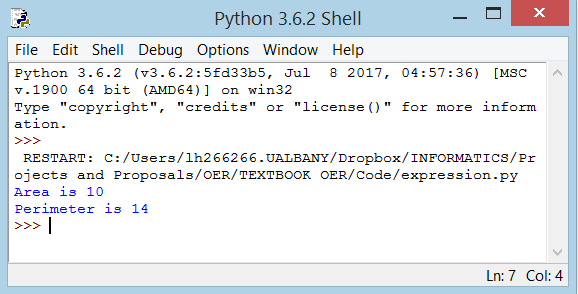Let us examine how this program works.

 Python Statement Explanation `length = 5` `width = 2` The `length` and `width` of the rectangle are stored in variables by the same name. They are each assigned an integer value. We use these to calculate the `area` and `perimeter` of the rectangle with the help of expressions. `area = length * width` `print('Area is', area)` We store the result of (assign) the expression `length * width` in the variable `area` and then print it using the `print` function. `print('Perimeter is', 2 * (length + width))` In this print statement, we directly use the value of the expression` 2 * (length + width)` in the print function. Also, notice how Python prints the output in a readable format even though we have not specified a space between `'Area is'` and the variable area (by adding a comma Python knows to separate the output as individual ‘words’, like you would in a sentence).

# Input/Process/Output Pattern

Recall from our previous Unit the example input-process-output diagram for the algorithm we called find_max.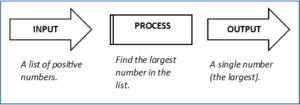Figure 20: Input-Process-Output for Finding the Largest Number

We can represent any solution to a computational problem using this pattern of identifying the input (the data we are given) and then the general process that must be completed to get the desired output.

We have used Python statements invoking the print function to display a string of characters (i.e. the “message”).

`print("This is a simple Python program")`

For starters, the built-in function print() will be used to print the output for our programs.

`name = input("Please enter your name: ")`

There is also a built-in function in Python for getting input from the user:

A sample run of this script in IDLE’s Python Shell would pop up a window like this: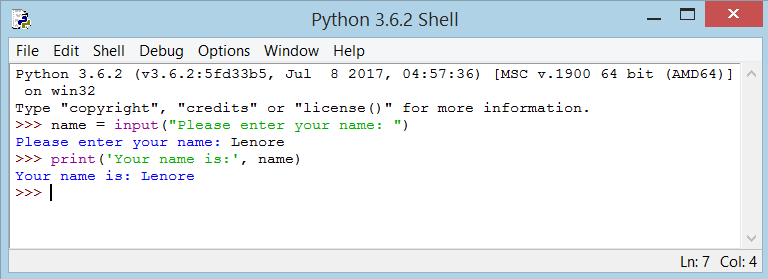Figure 21: Using the Built-in Function input()

The user of the program can enter the name and click OK (the Enter key), and when this happens the text that has been entered is returned from the `input` function, and in this case assigned to the variable `name`.

Even if you asked the user to enter their age, you would get back a string like `"17"`. It would be your job, as the programmer, to convert that string into an integer or a float value.

# Type Converter Functions

Here we’ll look at three more built-in Python functions, `int()`, `float()` and `str()`, which will (attempt to) convert their arguments into data types int, float and str respectively. We call these type converter functions.

The int function can take a floating point number or a string, and turn it into an int. For floating point numbers, it discards the decimal portion of the number — a process we call truncation towards zero on the number line. For example:

`>>> int(3.14)`
3
`>>> int(3.9999)     # This doesn't round to the closest int!`
3
`>>> int(3.0)`
3
`>>> int(-3.999)     # Note that the result is closer to zero`
`-3`
`>>> int(minutes / 60)`
`10`
`>>> int("2345")     # Parse a string to produce an int`
`2345`
`>>> int(17)         # It even works if arg is already an int`
`17`
`>>> int("23 bottles")`

This last type conversion case doesn’t look like a number — what do we expect?

```Traceback (most recent call last): File "<interactive input>", line 1, in <module></code ValueError: invalid literal for int() with base 10: '23 bottles'```

The type converter float() can turn an integer, a float, or a syntactically legal string into a float:

`>>> float(17)`
`17.0`
`>>> float("123.45")`
`123.45`

The type converter str() turns its argument into a string:

`>>> str(17)`
`'17'`
`>>> str(123.45)`
`'123.45'`

If you are not sure what class a value falls into (i.e. unsure whether a value is an integer, a float or a string), Python has a built-in function called type which can tell you.

`type('hello')`
`<class 'str'>`
`>>> type(29)`
`<class 'int'>`
`>>> num = 89.32`
`>>> type(num)`
`<class 'float'>`

# Python’s Standard Library

As mentioned in Unit #1, Python has an extensive Standard Library which is a collection of built-in modules, each providing specific functionality beyond what is included in the “core” part of Python. A Python module is simply a file that contains Python code. The name of the file dictates the name of the module; for example, a file named math.py contains the functions available from the standard math module. We will explore this module (math) here.

### Math Module and Math Functions

Python has a math module that provides most of the familiar mathematical functions. Before we can use the module, we have to import it:

>>> import math

This statement creates a module object named math. The following is a partial list of the functions which are provided by this module.

• `math.trunc(x)`: Returns the float value x truncated to an integer value.
• `math.sqrt(x)`: Returns the square root of x.
• `math.pow(x, y)`: Returns x raised to the power y.
• `math.degrees(x)`: Converts angle x from radians to degrees.
• `math.radians(x)`: Converts angle x from degrees to radians.

Many math operations depend on special constants also provided by the math module.

• `math.pi`: The mathematical constant `π = 3.141592`….
• `math.e`: The mathematical constant `e = 2.718281`….

Some examples of using the math module functions (note: these functions require the library name followed by a period followed by the function name):

`import math`
`math.exp(5)                # returns 148.4131591025766`
`math.e**5                  # returns 148.4131591025765`
`math.sqrt(144)             # returns 12.0`
`math.pow(12.5, 2.8)        # returns 1178.5500657314767`
`math.pow(144, 0.5)         # returns 12.0`
`math.trunc(1.001)          # returns 1math.trunc(1.999) # returns 1`
`12*math.pi**2              #returns 18.4352528130723`

Additional useful mathematical built-in functions besides `float()` and `int()` include:

• `abs(x)`: Returns the absolute value of the number x..
• `round( x [, n] )`: returns x rounded to n digits from the decimal point (n is optional). If n is omitted it returns the nearest integer to its input.

More examples of math functions (no need to import the `math` module with these functions):

`round(80.23456, 2)` # returns 80.23
`round(100.000056, 3)` # returns 100.0
`abs(-45)` # returns 45
`abs(100.12)` # returns 100.12

# More on Strings

We have seen how to print strings and how to get a string as input from a user. We also saw how to ‘add’ strings (concatenate) and ‘multiply’ strings.

`>>> word1='fun'`
`>>> word2='times'`
`>>> word1 word2`
`'funtimes'`
`>>> word1*4'`
`funfunfunfun'`

Processing of data includes the manipulation of strings (which is data) to produce something (information) which is meaningful. For example we might be presented with a file of thousands of passwords in use at a privately owned company and we would like to determine which of these passwords are secure and those that are not secure.

Recall that a string is a merely sequence of characters. To determine if an individual password was secure or not we might want to look at the length of the password and the individual characters in the password, looking for characters such as uppercase, numeric, special characters, etc.

Strings are actually a type of sequence; a sequence of individual characters. The indexing operator (Python uses square brackets to enclose the index) selects a single character substring from a string:

`>>> pw = "abc123"`
`>>> char1 = pw`
`>>> print(char1)`
`b`

The expression `pw` selects character number 1 from `pw`, and creates a new string containing just this one character. The variable `char1` refers to the result. When we display `char1`, we get the second character in the string `pw`, the letter “b”. Computer scientists always start counting from zero. The letter at subscript position zero of `"abc123"` is a. So at position `` we have the letter b.

If we want to access the zero-eth letter of a string, we just place 0, or any expression that evaluates to 0, in between the brackets:

`>>> pw = "abc123"`
`>>> char1 = pw`
`>>> print(char1)`
`a`

The expression in brackets is called an index. An index specifies a member of an ordered collection, in this case the collection of characters in the string. The index indicates which one you want, hence the name. It can be any integer expression.

Note that indexing returns a string — Python has no special type for a single character. It is just a string of length 1.

The string method` len()`, when applied to a string, returns the number of characters in a string:

`>>> pw = "abc123"`
`>>> len(pw)`
`6`

At some point, you may need to break a large string (i.g a paragraph) down into smaller chunks, or strings. This is the opposite of concatenation which merges or combines strings into one.

To do this, you use the` split()` method. What it does is split or breakup a string and add the data to a list of separate ‘words’ using a defined separator.

`>>> sentence = "Python is an interpreted high-level programming language for general-purpose programming."`
`>>> sentence.split()`
`['Python', 'is', 'an', 'interpreted', 'high-level', 'programming', 'language', 'for', 'general-purpose', 'programming.']`
`>>> len(sentence.split())`
`10`

If no separator is defined when you call upon the function, whitespace will be used by default (as seen above). In simpler terms, the separator is a defined character that will be placed between each variable. For example:

`>>> numbers = "122,35,09,97,56"`
`>>> numbers.split(",")`
`['122', '35', '09', '97', '56']`
`>>> len(numbers.split(","))`
`5`

The string method `lower()` converts all lowercase characters in a string into uppercase characters and returns it.

`>>> title="The Earth, My Butt, and Other Big Round Things"`
`>>> title.lower()'the earth, my butt, and other big round things'`
`>>>`

Similarly, The string method `upper()` converts all uppercase characters in a string into lowercase characters and returns it.

`>>> title="Where the wild things are"`
`>>> title.upper()`
`'WHERE THE WILD THINGS ARE'`
`>>>`

# Unit Summary

• Explain the dependencies between hardware and software
• Describe the form and the function of computer programming languages
• Create, modify, and explain computer programs following the input/process/output pattern.
• Form valid Python identifiers and expressions.
• Write Python statements to output information to the screen, assign values to variables, and accept information from the keyboard.
• Read and write programs that process numerical data and the Python math module.
• Read and write programs that process textual data using built-in functions and methods.

From now on, we will assume that you have Python installed on your system. You should now be able to write, save and run Python programs at ease.

Now that you are a Python user, let us learn some more Python concepts.

We have seen how to use operators, operands and expressions – these are the basic building blocks of any program.

Next, we will see how to make use of these in our programs using statements.

We have seen how to use the three control flow statements – if , while and for along with their associated break and continue statements. These are some of the most commonly used parts of Python and hence, becoming comfortable with them is essential.

# Practice Problems

1. What is the result of each of the following:>>> “Python”
a.>>> “Strings are sequences of characters.”
b.>>> len(“wonderful”)
c.>>> “Mystery”[:4]
d.>>> “p” in “Pineapple”
e.>>> “apple” in “Pineapple”
f.>>> “pear” not in “Pineapple”
g.>>> “apple” > “pineapple”
h.>>> “pineapple” < “Peach”
2. Take the sentence: `All work and no play makes Jack a dull boy`. Store each word in a separate variable, then print out the sentence on one line using the `print` function.
3. Add parenthesis to the expression `6 * 1 - 2` to change its value from `4` to `-6`.
4. The formula for computing the final amount if one is earning compound interest is given on Wikipedia as this formula for compound interest: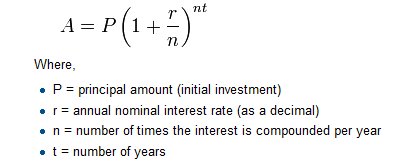Write a Python program that assigns the principal amount of \$10000 to variable `P`, assign to n the value 12, and assign to `r` the interest rate of 8%. Then have the program prompt the user for the number of years` t` that the money will be compounded for. Calculate and print the final amount after `t` years.
5. Evaluate the following numerical expressions on paper, then use the Python IDLE Shell to check your results:a.>>> 5 % 2
b.>>> 9 % 5
c.>>> 15 % 12
d.>>> 12 % 15
e.>>> 6 % 6
f.>>> 0 % 7
g.>>> 7 % 0
6. You look at the clock and it is exactly 2 pm. You set an alarm to go off in 51 hours. At what time does the alarm go off? (Hint: you could count on your fingers, but this is not what we’re after. If you are tempted to count on your fingers, change the 51 to 5100.)Write a Python program to solve the general version of the above problem. Ask the user for the time now (in hours), and ask for the number of hours to wait. Your program should output (print) what the time will be on the clock when the alarm goes off.
7. Write a program `find_hypot` which, given the length of two sides of a right-angled triangle, returns the length of the hypotenuse. (Hint: x ** 0.5 will return the square root.)
8. Practice using the Python IDLE Shell as a calculator:
1. Suppose the cover price of a book is \$24.95, but bookstores get a 40% discount. Shipping costs \$3 for the first copy and 75 cents for each additional copy. What is the total wholesale cost for 60 copies?
2. If I leave my house at 6:52 am and run 1 mile at an easy pace (8:15 per mile), then 3 miles at tempo (7:12 per mile) and 1 mile at easy pace again, what time do I get home for breakfast?
9. Enter the following statement, exactly, into the interactive Shell:`printt('What time is it?')`Is this a syntax error or a logic error?
10. Suppose that the math module of the Python Standard Library were imported. Write the Python statements to calculate the square root of four and print the answer.
11. What is the value of variables `num1` and `num2` after the following Python statements are executed?
1. `num = 0`
2. `new = 5`
3. `num1 = num + new * 2`
4. `num2 = num +  new * 2`
12. What is wrong with the following statement that attempts to assign the value ten to variable x?
1. `10 = x`
13. Classify each of the following as either a legal or illegal Python identifier:a. fred
b .if
c. 2x
d.-4
e. sum_total
f. sumTotal
g. sumtotal
h. While
i. x2
j. Private
k. public
l. \$16
m. xTwo
n. 10%
o. a27834
14. How is the value 2.45 x 10-5 expressed as a Python literal?
15. Given the following assignment:x = 2: Indicate what each of the following Python statements would print.
a. print(“x”)
b. print(‘x’)
c. print(x)
d. print(“x + 1”)
e. print(‘x’ + 1)
f.  print(x + 1)
16. Given the following assignments:
i1 = 2
i2 = 5
i3 = -3
d1 = 2.0
d2 = 5.0
d3 = -0.5
17. Evaluate each of the following Python expressions.
1.  i1 + i2
2. i1 / i2
3. i1 // i2
4. i2 / i1
5. i1 * i3
6. d1 + d2
7. d1 / d2
8. d2 / d1
9. d3 * d1
10. d1 + i2
11. i1 / d2
12. d2 / i1
13. i2 / d1
14. i1/i2*d1
18. What is printed by the following statement:
#print(5/3)
19. Consider the following program which contains some errors. You may assume that the comments within the program accurately describe the program’s intended behavior.# Get two numbers from the user
n1 = float(input()) # first number
n2 = float(input()) # second number
# Compute sum of the two numbers
print(n1 + n2) # third number
# Compute average of the two numbers
print(n1+ n2/2) # fourth number
# Assign some variables
d1 = d2 = 0 # fifth number
# Compute a quotient
print(n1/d1) # sixth number
# Compute a product
n1*n2 = d1 # seventh number
# Print result
print(d1) # eighth numberFor each line listed in the comments, indicate whether or not a syntax error, run-time error, or semantic error is present. Not all lines contain an error.
20. What is printed by the following code fragment?x1 = 2
x2 = 2
x1 += 1
x2 -= 1
print(x1)
print(x2)
21. Given the following definitions:
x = 3
y = 5
z = 7evaluate the following Boolean expressions:
x == 3
x < y
x >= y
x <= y
x != y – 2
x < 10
x >= 0 and x < 10
x < 0 and x < 10
x >= 0 and x < 2
x < 0 or x < 10
x > 0 or x < 10
x < 0 or x > 10
22. Given the following definitions:x =3
y = 3
b1 = true
b2 = false
x == 3
y < 3
evaluate the following Boolean expressions:
1. b2
2. not b1
3. not b2
4. b1 and b2
5. b1 or b2
6. x
7. y
8. x or y
9. not y

1. Dierbach, Charles. Introduction to Computer Science Using Python: A Computational Problem-solving Focus. Wiley Publishing, 2012, p9.
2. Dierbach, Charles. Introduction to Computer Science Using Python: A Computational Problem-solving Focus. Wiley Publishing, 2012, p10
3. Dierbach, Charles. Introduction to Computer Science Using Python: A Computational Problem-solving Focus. Wiley Publishing, 2012, pp.11-12.
4. Based on information from: https://www.softwaretestingmaterial.com/compiler-vs-interpreter/